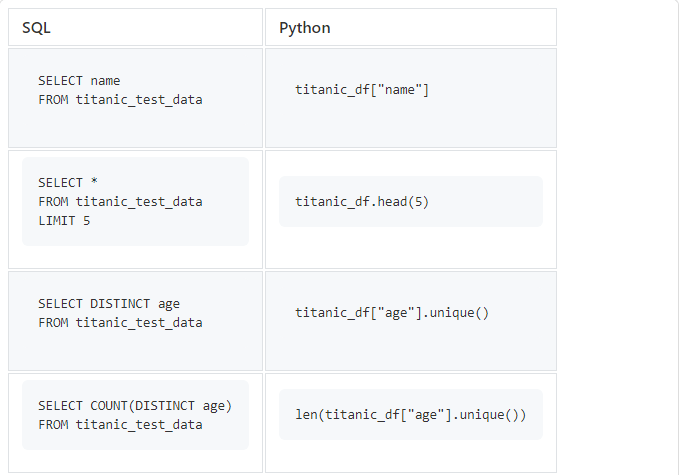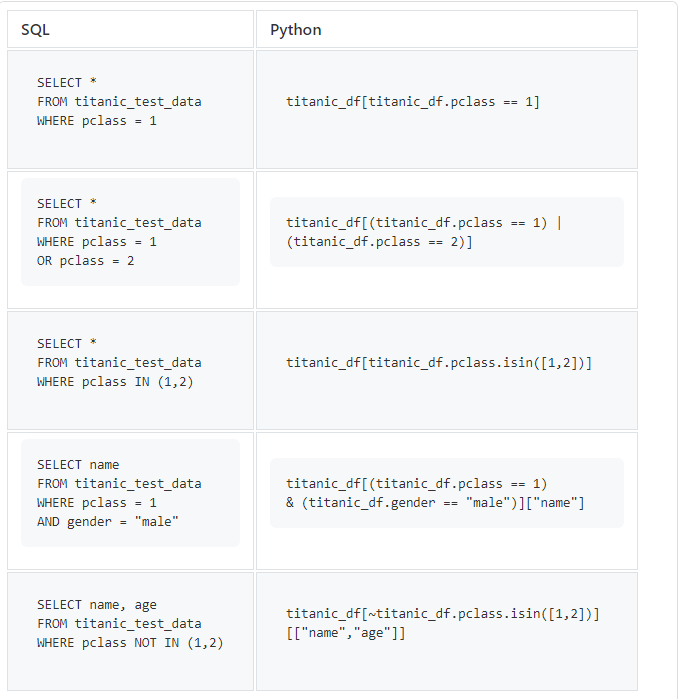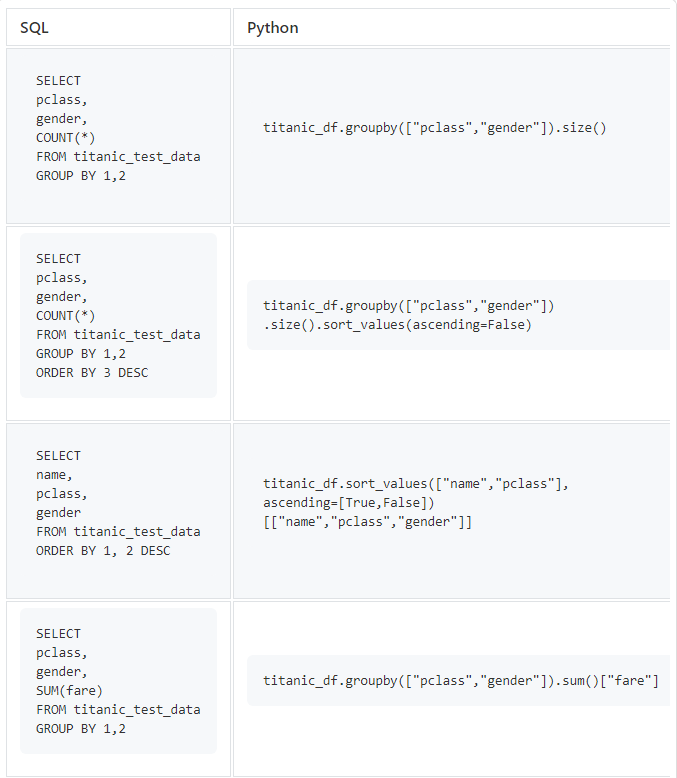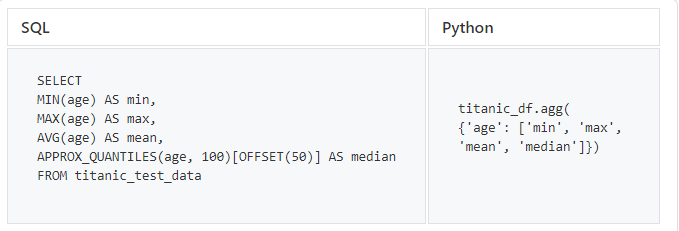# How to rewrite SQL queries in Python using Pandas

## 3 min

In this article, June Tao Ching described how to achieve the same result in Python as in SQL queries using Pandas. Before you is a translation, and original you can find the blog towardsdatascience.com.Photo from the site Unsplash… Author: Hitesh choudhary

### Getting the same result in Python as with an SQL query

Often, when working on the same project, we have to switch between SQL and Python. That being said, some of us are familiar with data manipulation in SQL queries, but not in Python, which hinders our efficiency and productivity. In fact, using Pandas, you can achieve the same result in Python as in SQL queries.

### Beginning of work

The Pandas package needs to be installed if it is not there.

``conda install pandas``

We will use the famous Dataset Titanic by Kaggle

After installing the package and downloading the data, we need to import it into our Python environment.We will use a DataFrame to store data. Various Pandas functions will help us manage this data structure.

### SELECT, DISTINCT, COUNT, LIMIT`titanic_df["age"].unique()` will return an array of unique values, so we’ll have to use `len()`to count their number.

### SELECT, WHERE, OR, AND, IN (SELECT with conditions)

After the first part, you learned how to explore a DataFrame in simple ways. Now let’s try to do this with some conditions (this is the operator `WHERE` in SQL).If we only want to select specific columns from the DataFrame, we can do so with an extra pair of square brackets.

Note: if you select multiple columns you need to put an array `["name","age"]` inside square brackets.

`isin()` works exactly the same as `IN` in SQL queries. To use `NOT IN`, in Python we need to use negation `(~)`

### GROUP BY, ORDER BY, COUNT

`GROUP BY` and `ORDER BY` are also popular SQL statements for data mining. Now let’s try using them in Python.If we want to sort only one COUNT column, then we can simply pass a boolean value to the method `sort_values`… If we are going to sort several columns, then we must pass an array of boolean values ​​to the method `sort_values`

Method `sum()` will give sums for each of the columns in the DataFrame, which can be aggregated numerically. If we only want a specific column, then we need to specify the column name using square brackets.

### MIN, MAX, MEAN, MEDIAN

Finally, let’s try some of the standard statistical functions that are important when exploring data.SQL does not contain operators that return the median value, so we use BigQuery to get the median value of the age column. `APPROX_QUANTILES `

In Pandas, the aggregation method is `.agg()` also supports other features like `sum`

Now you have learned rewrite SQL queries in Python with Pandas… Hope you find this article helpful.

All code can be found in my repository Github